Impulse Momentum Exam2 and Problem Solutions

Impulse Momentum Exam2 and Problem Solutions

1. Objects shown in the figure collide and stick and move together. Find final velocity objects.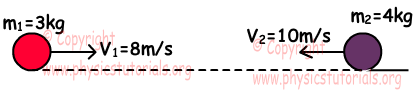Using conservation of momentum law;

m1.V1+m2.V2=(m1+m2).Vfinal

3.8+4.10=7.Vfinal

64=7.Vfinal Vfinal=9,14m/s

2. 2kg and 3kg objects slide together, and then they break apart. If the final velocity of m2 is 10 m/s,

a) Find the velocity of object m1.

b) Find the total change in the kinetic energies of the objects.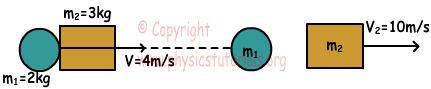a) Using conservation of momentum law;

(m1+m2).V=m1.V1+m2.V2

5.4=30+2.V1

V1=-5m/s

b) EKinitial=1/2/m1+m2).V2

EKinitial=1/2.5.16=40joule

EKfinal=1/2.2.52+1/2.3.102

EKfinal=175 joule Change in the kinetic energy is =175-40=135 joule

3. As shown in the figure below, object m1 collides stationary object m2.Find the magnitudes of velocities of the objects after collision. (elastic collision)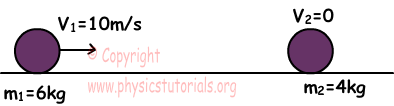In elastic collisions we find velocities of objects after collision with following formulas;

V1'=(m1-m2)/(m1+m2).V1

V2'=(2m1/m1+m2).V1

m1=6kg, m2=4kg, V1=10m/s

V1'=(6-4/6+4).10=2m/s

V2'=(2.6/6+4).10=12m/s

4. Momentum vs. time graph of object is given below. Find forces applied on object for each interval.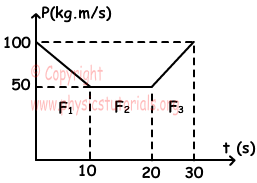F.Δt=ΔP

F=ΔP/Δt

Slope of the graph gives us applied force.

I. Interval: F1=P2-P1/10-0=-50/10=-5N

II. Interval: F2=50-50/10=0

III. Interval: F3=100-50/10=5N

5. A box having mass 0,5kg is placed in front of a 20 cm compressed spring. When the spring released, box having mass m1, collide box having mass m2 and they move together. Find the velocity of boxes.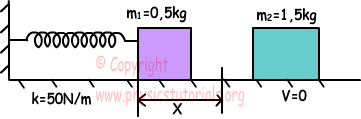Energy stored in the spring is transferred to the object m1.

1/2.k.X2=1/2.mV2

50N/m.(0,2)2=0,5.V2

V=2m/s

Two object do inelastic collision.

m1.V1=(m1+m2).Vfinal

0,5.2=2.Vfinal

Vfinal=0,5m/s

Author: